#DotNetCsg 1.0.1

.NET Standard 2.0
`dotnet add package DotNetCsg --version 1.0.1`
`NuGet\Install-Package DotNetCsg -Version 1.0.1`
This command is intended to be used within the Package Manager Console in Visual Studio, as it uses the NuGet module's version of Install-Package.
`<PackageReference Include="DotNetCsg" Version="1.0.1" />`
For projects that support PackageReference, copy this XML node into the project file to reference the package.
`paket add DotNetCsg --version 1.0.1`
`#r "nuget: DotNetCsg, 1.0.1"`
#r directive can be used in F# Interactive and Polyglot Notebooks. Copy this into the interactive tool or source code of the script to reference the package.
```// Install DotNetCsg as a Cake Addin

// Install DotNetCsg as a Cake Tool
#tool nuget:?package=DotNetCsg&version=1.0.1```

## DotNetCsg

DotNetCsg is a Constructive Solid Geometry (CSG) modeling library that provides 3D solid primitives and operators to manipulate them.

This library targets .NET Standard 2.0 and has no dependencies, so you should be able to deploy it anywhere .NET is.

If you're new to CSG:

• The CSG Wikipedia page has some good information (with pictures too).
• Try out OpenSCAD. It has a friendly interface and gives immediate results.

Try out this library if:

• You're comfortable learning CSG in a .NET environment (Visual Studio, C#, etc -- the stuff you use every day).
• Your new or existing .NET application needs CSG functionality.

### Example

``````using Csg;
using static Csg.Solids;

var wiki = Difference(
Intersection(
Cube(size: 1.4, center: true),
Sphere(r: 1, center: true)
),
Union(
rod,
rod.RotateX(90),
rod.RotateZ(90)
)
);

using (var fs = File.Create(\$"wiki.stl"))
using (var wr = new StreamWriter(fs))
{
wiki.WriteStl("wiki", wr);
}
``````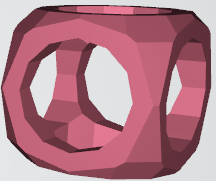### Snippets

#### Namespaces

``````// All types in this library are in the Csg namespace
using Csg;

// Use this for simpler calls to solids and operations
using static Csg.Solids;
``````

#### Basic Shapes

``````// Create a basic cube
var cube = Cube(size: 1, center: true);

// Create a basic sphere
var sphere = Sphere(r: 0.5, center: true);

// Create a basic cylinder
var cylinder = Cylinder(r: 0.5, h: 1, center: true);
``````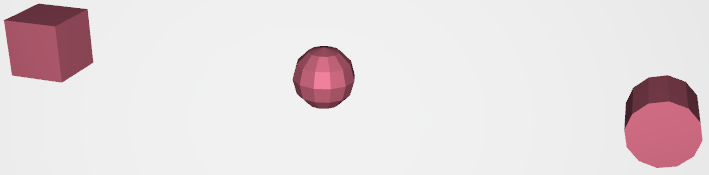#### Moving (Translate)

``````// Create a small cube in the center
var smallCube = Cube(size: 0.4, center: true);

// Have the cube to the right (x-axis)
var moveCube = cube.Translate(x: +2);

// Have a sphere forward (y-axis)
var moveSphere = sphere.Translate(y: +2);

// Have a cylinder up (z-axis)
var moveCylinder = cylinder.Translate(z: +2);
``````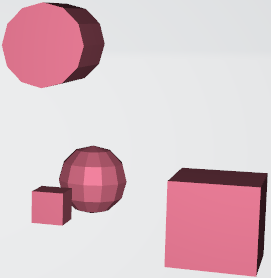#### Operations

``````var offsetSphere = sphere.Translate(x: 0.5, y: 0.5, z: 0.5);

// Union combines the shapes together, even if there's no shared area
var union = Union(cube, offsetSphere);

// Difference removes the second shape, taking the shared area from the first shape as well
var difference = Difference(cube, offsetSphere);

// Intersection keeps on the shared area of both shapes, if there's no shared area then it returns empty space
var intersection = Intersection(cube, offsetSphere);

// Note that you can also call the operations via member functions with no difference to the object returned
//var union = cube.Union(offsetSphere);
//var difference = cube.Subtract(offsetSphere);
//var intersection = cube.Intersect(offsetSphere);
``````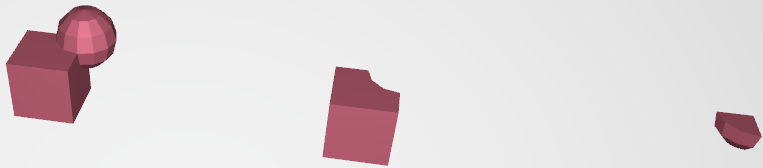#### Example: Vintage Barbell

``````var bar = Cylinder(r: 0.025, h: 2.1, center: true);

var weight = Sphere(r: 0.2, center: true);

// Note that we're using the same weight object more than once
// Every function that returns a Solid will not modify the original object
// So you can create your geometry and then place it in different locations

var barbell = Union(bar,
weight.Translate(y: -0.9),
weight.Translate(y: +0.9)
);
``````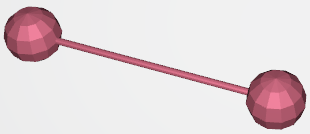#### Example: Pipe

``````var outer = Cylinder(r: 1, h: 4, center: true);
var inner = Cylinder(r: 0.95, h: 4, center: true);

var pipe = Difference(outer, inner);
``````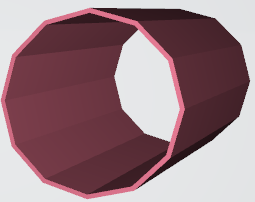#### Writing shape to a file

``````using (var fs = File.Create(\$"cube.stl"))
using (var wr = new StreamWriter(fs))
//using (var wr = new BinaryWriter(fs)) // Note you can also use BinaryWriter to write as an STL binary file
{
cube.WriteStl("cube", wr);
}
``````

### History

This library is a fork of hypar-io/Csg, which is a fork of praeclarum/Csg, which is a manual port of OpenJsCad's csg.js.

My reason for this fork is to get the changes from hypar-io/Csg into a NuGet package, and to build up some documentation around the library (but it's quite easy to use without documentation).

### Building

To build, ensure that you have the latest .NET SDK installed.

Product Compatible and additional computed target framework versions.
.NET net5.0 was computed.  net5.0-windows was computed.  net6.0 was computed.  net6.0-android was computed.  net6.0-ios was computed.  net6.0-maccatalyst was computed.  net6.0-macos was computed.  net6.0-tvos was computed.  net6.0-windows was computed.  net7.0 was computed.  net7.0-android was computed.  net7.0-ios was computed.  net7.0-maccatalyst was computed.  net7.0-macos was computed.  net7.0-tvos was computed.  net7.0-windows was computed.  net8.0 was computed.  net8.0-android was computed.  net8.0-ios was computed.  net8.0-maccatalyst was computed.  net8.0-macos was computed.  net8.0-tvos was computed.  net8.0-windows was computed.
.NET Core netcoreapp2.0 was computed.  netcoreapp2.1 was computed.  netcoreapp2.2 was computed.  netcoreapp3.0 was computed.  netcoreapp3.1 was computed.
.NET Standard netstandard2.0 is compatible.  netstandard2.1 was computed.
.NET Framework net461 was computed.  net462 was computed.  net463 was computed.  net47 was computed.  net471 was computed.  net472 was computed.  net48 was computed.  net481 was computed.
MonoAndroid monoandroid was computed.
MonoMac monomac was computed.
MonoTouch monotouch was computed.
Tizen tizen40 was computed.  tizen60 was computed.
Xamarin.iOS xamarinios was computed.
Xamarin.Mac xamarinmac was computed.
Xamarin.TVOS xamarintvos was computed.
Xamarin.WatchOS xamarinwatchos was computed.
Compatible target framework(s)
• #### .NETStandard 2.0

• No dependencies.

### NuGet packages

This package is not used by any NuGet packages.

### GitHub repositories

This package is not used by any popular GitHub repositories.